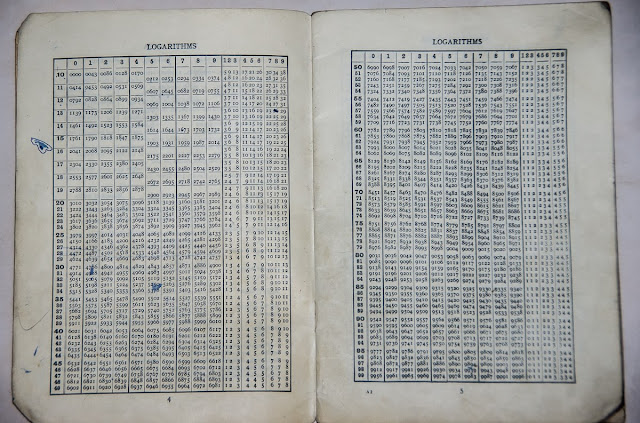## Tuesday, February 5, 2019Logarithm table

#### Nonlinear model

How to solve:

Nonlinear model
\begin{align} \min & \sum_i \color{DarkRed} x_i\\ & \sum_i \color{DarkBlue} a_i \log \color{DarkRed} x_i \ge \color{DarkBlue} c \\ & \color{DarkRed}x_i \in \{\color{DarkBlue}b_1,\color{DarkBlue}b_2,\dots,\color{DarkBlue}b_m\} \end{align}

where we have $$n$$ variables $$x_i$$, $$i=1,\dots,n$$. This model looks nonlinear because of the logarithms.

#### Linear model

The condition $$x_i \in \{b_1,b_2,\dots,b_m \}$$ is essentially a table lookup. This can be modeled using binary variables: $y_{i,j} = \begin{cases} 1 & \text{if x_i=b_j}\\ 0 & \text{otherwise}\end{cases}$ With this definition we can write \begin{align} & x_i = \sum_j y_{i,j} b_j \\ & \sum_j y_{i,j}=1 && \forall i\\ & y_{i,j} \in \{0,1\} \end{align}

Maybe somewhat surprisingly, we can also calculate $$\log x_i$$ using these $$y_{i,j}$$: $\mathit{logx}_i = \sum_j y_{i,j} \log(b_j)$ This is very fortunate! We get the logs essentially for free! So our complete model can look like:

Linear model
\begin{align} \min & \sum_i \color{DarkRed} x_i\\ & \color{DarkRed} x_i = \sum_j \color{DarkRed} y_{i,j} \color{DarkBlue} b_j \\ & \color{DarkRed}{\mathit{logx}}_i = \sum_j \color{DarkRed} y_{i,j} \log(\color{DarkBlue} b_j) \\ & \sum_j \color{DarkRed} y_{i,j}=1 && \forall i \\ & \sum_i \color{DarkBlue} a_i \color{DarkRed}{\mathit{logx}}_i \ge \color{DarkBlue} c \\ & \color{DarkRed}y_{i,j} \in \{0,1\} \end{align}

This model is now completely linear and we can use a standard Mixed Integer Programming (MIP) solver to solve it.

#### More compact models

If we want we can save a few variables and equations by substituting out the variable $$\mathit{logx}_i$$.  It would make the model a bit more difficult to read: $\sum_{i,j} a_i \log(b_j) y_{i,j} \ge c$ may not be completely obvious. Furthermore we can substitute out the variables $$x_i$$ and just keep the $$y_{i,j}$$ variables. Of course, you would need to recover, after the solve, the values for $$x_i$$ from the optimal values $$y_{i,j}^*$$. That model would be very compact, but would need some explanation, as it is not completely self-explanatory.

#### Polynomial constraints?

In  someone suggested to form the polynomial equations $(x_i-b_1)(x_i-b_2)\cdots(x_i-b_m)=0$ and solve as a Nonlinear Programming (NLP) problem. This is surely not a good approach. If this was really a great idea, we would no longer need MIP solvers! Instead of binary variables, we just could form $x(x-1)=0$ For an integer variable $$x \in \{0,1,\dots,n\}$$ we could write $x(x-1)(x-2)\cdots(x-n)=0$ The reason why this does not work, is that we create a very difficult non-convex problem. Unless you use a global solver, an NLP solver will just get stuck in a local optimum (or may not find a feasible solution). Don't throw away your MIP solver yet.

#### Final remarks

Using random data I was able to solve a problem with $$n=m=100$$ in a few seconds. Of course this depends on the data: other instances may take more time.

This discussion reminds me a little bit of linear regression problems. In many cases, non-linearities appear in the data only, and although the regression model looks non-linear, it can actually be handled by linear regression approaches.

#### References

1. How to solve the following optimization problem of mathematical programming?, https://math.stackexchange.com/questions/3090493/how-to-solve-the-following-optimization-problem-of-mathematical-programming
2. MIP Modeling: from Sudoku to KenKen via Logarithms, https://yetanothermathprogrammingconsultant.blogspot.com/2016/10/mip-modeling-from-sudoku-to-kenken.html. The model to solve KenKen puzzles uses a similar approach.